A function of an angular frequencydefining a weight function used in the non-parametric estimation of the spectral density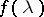of a stationary stochastic process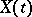by smoothing the periodogram constructed from the observed data of the process. As an estimator of the value of the spectral density at a pointone usually takes the integral with respect to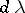of the product of the periodogram atand an expression of the form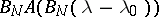. Here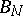is a real number and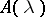is a fixed function of the frequency which takes its greatest value atand is such that its integral overis equal to one. This function is usually called a spectral window generator, while the term "spectral window" is used for the function. The width of the spectral window is, and depends on the sizeof the sample (that is, on the length of the observed realization of the process) and tends to zero as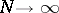(but more slowly than). The Fourier transform of the spectral window (and in the case of discrete time, when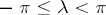, the set of its Fourier coefficients) is called the lag window of an estimator of the spectral density. It defines a weight function of a discrete or continuous argument (depending on whetheris discrete or continuous), by which one must multiply the sample auto-correlations evaluated from the given sample to make the Fourier transform of the resulting product coincide with the desired estimator of the spectral density (cf. Spectral density, estimator of the).